Shear Strength of Soil

# Shear Strength of Soil - Notes | Study Civil Engineering SSC JE (Technical) - Civil Engineering (CE)

 1 Crore+ students have signed up on EduRev. Have you?

Chapter 9
Shear Strength of Soil

SHEAR STRENGTH
Shear strength of a soil is the capacity of the soil to resist shearing stress. It can be defined as the maximum value of shear stress that can be defined as the maximum value of shear stress that can be Mobilized within a soil mass.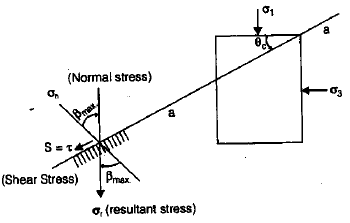-Plane a -a is critical plane
c = Angle of critical plane (a – a)
1 and σ3 are stresses on given planes

(i)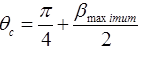where, βmax = Angle between resultant stress and normal stress on critical plane=Friction angle of soil = ∅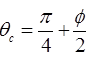for clay, ∅= 0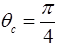(ii)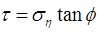, for sands or Φ -soil.

(iii)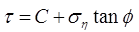, for C- Φ soil.

(iv)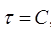, for C-soil (clays).

(v)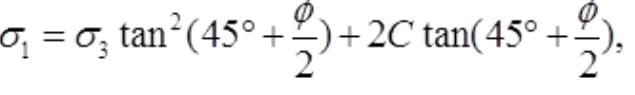for C-∅  soil.

(vi)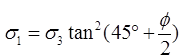, for Φ soil.
(vii) σ1= 2C , for C– soil.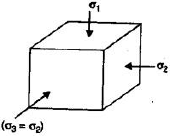MOHR COULOMBS THEORY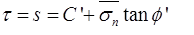where, C’ = Effective Cohesion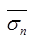= Effective normal stress and ∅' = Effective friciton angle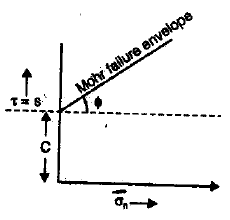DIRECT SHEAR TEST: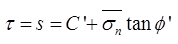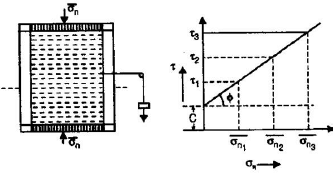UNCONFINED COMPRESSION TEST

-qu = (σ1)f
where, qu = unconfined compressive strength.
Here, σ3= 0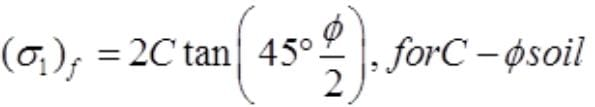- (σ1)f =2C , for C-soil
-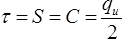for clay’s or C-soil.

VANE SHEAR TEST:
-It is suitable for sensitive clays.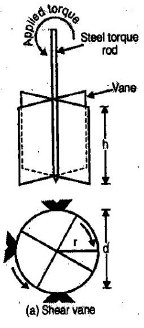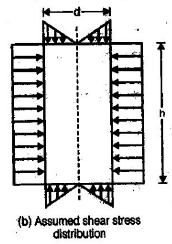Lab size Field Size Height of vane (H) 20 mm 10 to 20 cm Dia of vane (D) 12 mm 5 to 10 cm Thickness of vane (t) 0.5 to 0.1 mm 2 to 3 cm

Shear Strength: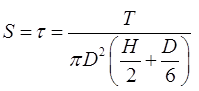-When top and bottom of vanes both pertain in shearing.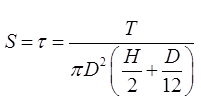-When only bottom of vanes pertain in shearing.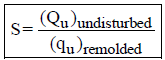where Sf = Sensitivity

PORE PRESSURE PARAMETER: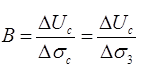where,
B = Pore pressure parameter
ΔUc = Change in pore pressure due to in crease in cell pressure
Δσ= Δσ3 = Change in cell pressure.
-0 ≤ B ≤ 1
-B = 0, for dry soil.
-B = 1, for saturated soil.

(ii)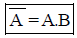where A = Pore pressure parameter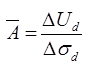where ,
ΔUd = Change in pore pressure due to deviator stress.
Δσd = Change in deviator stress

(iii) ΔU=ΔUc +ΔUd
ΔU = change in pore pressure
(iv)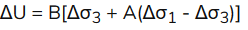The document Shear Strength of Soil - Notes | Study Civil Engineering SSC JE (Technical) - Civil Engineering (CE) is a part of the Civil Engineering (CE) Course Civil Engineering SSC JE (Technical).
All you need of Civil Engineering (CE) at this link: Civil Engineering (CE)

## Civil Engineering SSC JE (Technical)

2 videos|122 docs|50 tests
 Use Code STAYHOME200 and get INR 200 additional OFF

## Civil Engineering SSC JE (Technical)

2 videos|122 docs|50 tests

Track your progress, build streaks, highlight & save important lessons and more!

,

,

,

,

,

,

,

,

,

,

,

,

,

,

,

,

,

,

,

,

,

;